# 论文理解 - ImageNet Classification with Deep Convolutional Neural Networks

[AlexNet - Paper]

[原文地址]

Li的学生。下面这幅图是模型的架构图（五个卷积后接三个全连接，最后是处理1000个元素的feature
map的softmax，但softmax没画出来。后文会具体说明）：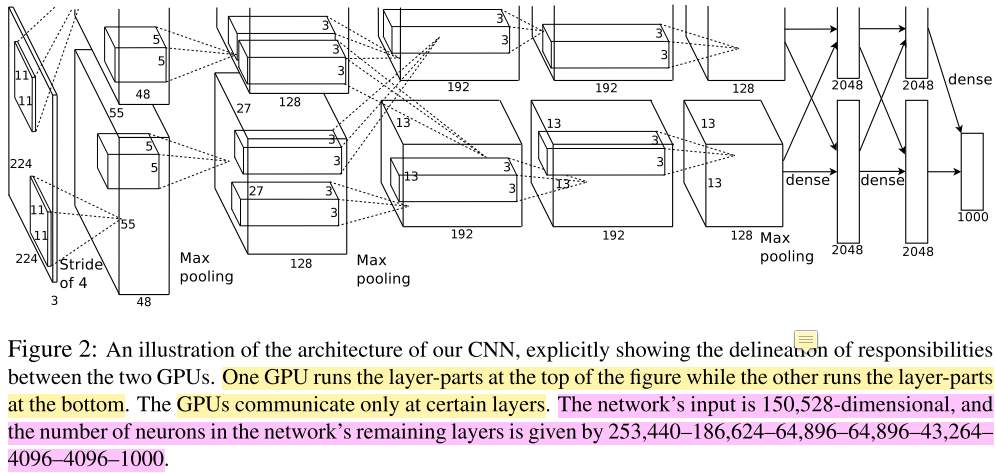1. 收敛/训练速度加快，用了非饱和神经元（ReLU，即线性矫正单元）。ReLU是一种非线性神经元（当输入x大于0，则输出为x；输入x小于0，则输出0）较之前的激活函数计算速度更快，收敛也更快；

2. 模型并行。文中并没写是模型并行，但我看介绍认为是一种（有数据并行的）模型并行。训练过程用了两个GTX580 GPU 3GB（一个gpu无法cover住），除了第三层卷积、最后的两个全连接层（两个GPU将全连接拆成各自两部分）和 Softmax（汇聚到单个GPU处理），其余部分处理可以看作两个GPU的数据并行（AlexNet架构图的上部分和下部分对应两个GPU各自的处理流程，单GPU的话，就是 group=2 这个可以看后文中通过netscope可视化出的网络结构）。相比单GPU没加快多少，同时某些层两个GPU共享参数，可以减少显存占用这样以便在一次参数更新放更多的图片加入训练。发现双卡比单卡top1和top5的error有下降（我认为是作者忘了改SGD学习率导致，先跑one-gpu net再跑two-gpu net）；

3. 正则化——数据扩增和dropout。关于数据增强，我的理解是：有变化才能找到规律。只要保证数据多样性的同时，同样的图像有些许的变化（不aug过头），这样网络的权重才能学到在变化中，输入到输出的映射本质，学习出/建立起鲁棒的网络权重/参数。没dropout模型训练很快会过拟合，加上后double了收敛时间（iteration次数）。但这一项没有做ablation study（或许做了文中没说），感觉这个dropout不convincing；
4. 性能state of art。top-1和top-5错误率分别为37.5%和17.5%。相比第二 top-5 错分率是26.2%。我觉得除以上外，性能提升的一个重要因素是做了数据扩增（data augmentation），产生更diverse的数据来拟合模型。

1. 任务背景
1.1 Model Capacity/Depth
1.2 ImageNet
2. Architecture
2.2 多卡训练
2.3 Local Response Normalization
2.4 Overlapping Pooling
3. Reducing Overfitting
3.1 Data Augmentation
3.2 Dropout
4. Details of learning
5. Feature Extractor
6. 参考


1. Local Response Normalization 的 backprop 是怎么做的（后文给出了caffe的代码）；
2. data augmentation 的 Fancy PCA 怎么算一张三通道图像的特征值和特征向量的（后文给出了cuda-convnet代码位置）？

## 1. 任务背景

### 1.1 Model Capacity/Depth

Thus, compared to standard feedforward neural networks with similarly-sized layers, CNNs have much fewer connections and parameters and so they are easier to train, while their theoretically-best performance is likely to be only slightly worse.

Our final network contains five convolutional and three fully-connected layers, and this depth seems to be important: we found that removing any convolutional layer (each of which contains no more than 1% of the model’s parameters) resulted in inferior performance.

our network’s performance degrades if a single convolutional layer is removed. For example, removing any of the middle layers results in a loss of about 2% for the top-1 performance of the network. So the depth really is important for achieving our results.

our results have improved as we have made our network larger and trained it longer.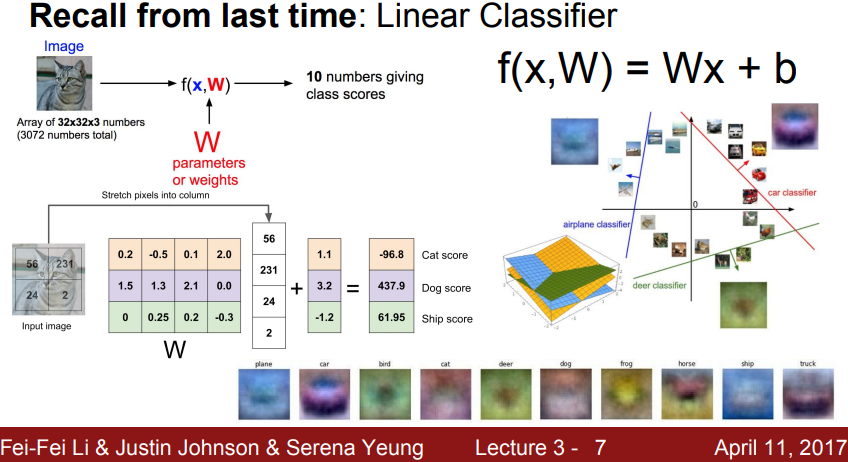### 1.2 ImageNet

ImageNet包含22000个类别的1500万张图像（主要标注任务由项目负责人 FeiFei Li 组织完成，大部分标注任务使用了亚马逊的众包项目完成）。因为ImageNet这个项目和FeiFei Li等人的不懈努力，有一个名叫ILSVRC（ImageNet Large Scale Visual Recognition Challenge）的年度比赛，作为 Pascal Visual Object Challenge 的一部分。但ILSVRC只用了ImageNet数据集中的一个子集数据：包含1000类，每类大概1000张图像，训练集有120万张图像、验证集 5 万张图像以及 15 万张用来测试的图像。分类任务的模型性能习惯用top-1和top-5两个错误率进行不同模型的比较。

## 2. Architecture• 输入层：作者说网络输入是 150528 维度，也就是输入图像的长、宽和通道数的乘积$（224×224×3=150528）$$（224×224×3=150528）$。接下来经过第一层卷积操作，第一层卷积操作的具体参数量为 11712；

• 卷积层1：卷积核宽和高为11，即11×11，网络架构图上有上下两路，两路feature map通道数的总和96，等同于 output channel 的数量，这个结论我是根据CS231n中的下面这张图得出的（可以看到有两个卷积核 w0和 w1，而且每个卷积核的维度都是 3×3×3 的带深度的三维结构，需要注意的是：每个卷积核处理上一层输入的一张图三个通道，那么卷积核也是三维的，同时每个卷积核还有1个偏执单元，那么 2 卷积核有2个bias，总参数量为：$3×3×3×2+2=56$$3×3×3×2+2=56$，那么总共的卷积参数大小为：$\left(11×11×3×48+48\right)×2=34944$$(11×11×3×48+48)×2=34944$。主要在于这里是上下两路，单路是 $11×11×3×48+48=17472$$11×11×3×48+48=17472$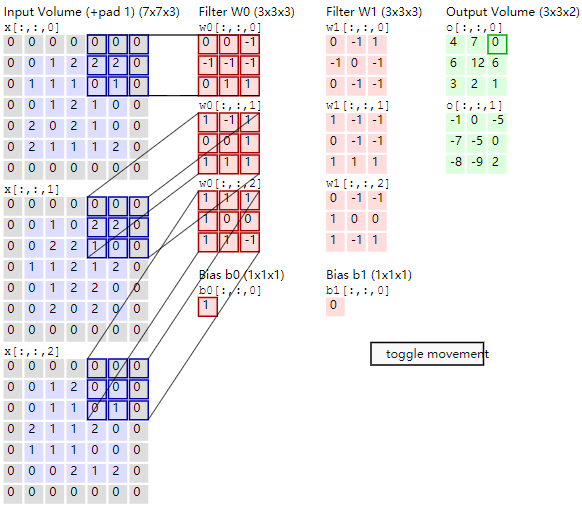• 卷积层1得到的feature map：第一个卷积层后得到 feature map，由两个48通道的 feature map合起来看，相当于算这个立方体的体积。总维度为 $55×55×48×2=290400$$55×55×48×2=290400$（作者图注写的是253400是错的）。feature map的计算是根据上一层（输入）的长宽减去filter的长宽，加上2倍的padding的和，除以stride 4，最后加1，即 $\left(224–11+2×0\right)÷4+1=54.25$$(224–11+2×0)÷4+1=54.25$，会发现这里不是 55 且带小数，CS231n课程中有下面一段说Alex当时可能是在 227 的图上做的（但是下面这段话说Alex做了padding，我觉得如果224的图要padding得到55的话，要这么算：$\left(224–11+2×1.5\right)÷4+1=55$$(224–11+2×1.5)÷4+1=55$，也就是说padding=1.5），即 $\left(227–11+2×0\right)÷4+1=55$$(227–11+2×0)÷4+1=55$

As a fun aside, if you read the actual paper it claims that the input images were 224×224, which is surely incorrect
because (224 – 11)/4 + 1 is quite clearly not an integer. This has confused many people in the history of ConvNets and little is known about what happened. My own best guess is that Alex used zero-padding of 3 extra pixels that he does not mention in the paper.

from CS231n blog

• norm1层：norm就是 local response normalization，基于局部小区域的像素值强度归一化（后面介绍），对feature map大小没有影响；

• pool1层（pool：max；kernel size：3；stride：2）：上一层卷积feature map维度（把两个支路一起看）为：$W=55,H=55,D=96$$W=55,H=55,D=96$, 分别表示宽度、高度、深度（channel数）。经过pool后的结果这么算：$W1=\left(W-F\right)/S+1;H1=\left(H–F\right)/S+1;D2=D1$$W1=(W−F)/S+1; H1=(H–F)/S+1; D2=D1$，其中 $F$$F$$S$$S$ 分别是两个pool的超参数池化空间扩展（spatial extent，相当于池化的kernel）和池化步幅（Stride）。那么得到的新的 feature volume的长、宽、深（通道数）为 $W1=27;H1=27;D1=96$$W1=27; H1=27; D1=96$，整体规模为 $\left[\left(55-3\right)/2+1\right]×\left[\left(55–3\right)/2+1\right]×96=69984$$[(55−3)/2+1]×[(55–3)/2+1]×96=69984$

• 卷积层2：权重规模为（拆成两路算）：$\left(5×5×48×128+128\right)×2=307456$$(5×5×48×128+128)×2=307456$。其实有个很基础的公式（也带计算bias）：$\mathrm{#}inputchannel×{\mathrm{#}kernelsize}^{2}×\mathrm{#}outputchannel+\mathrm{#}outputchannel$${\#input channel}×{\#kernel size}^2×{\#output channel} + {\#output channel}$，这里是双路GPU所有每一路算各自的，最后乘以2.

• 卷积层2得到的feature map：$27×27×128×2=186624$$27×27×128×2=186624$

• norm2：略，186624；

• pool2：$\left[\left(27–3\right)/2+1\right]×\left[\left(27–3\right)/2+1\right]×128×2=43264$$[(27–3)/2+1]×[(27–3)/2+1]×128×2=43264$

• 卷积层3：权重规模为：$\left(3×3×128×192×2+192×2\right)×2=885504$$(3×3×128×192×2+192×2)×2=885504$，但是我根据netscope的网络结构（没有group，注意没有分支）图算出来的权重规模为：$3×3×256×384+384=885120$$3×3×256×384+384=885120$。后来发现这个885504（前一个）算的不对，因为文中说到：the kernels of layer 3 take input from all kernel maps in layer 2，所以这里就把两条支路当做一条路，前一层上下两路的feature map当做一起的，后面得到的上下两路feature map 一当做一起的。前者多出来一个384，是因为多了一个GPU产生的bias数目。或许因此，two-gpu net 比 one-gpu net的性能好（我的猜测后文是作者又尝试了非GTX580的大显存卡吧，当然不排除作者减小 input shape 和模型中的 kernel size 来训练 one-gpu net。这里作者也没讲清）；

• 卷积层3得到的 feature map：$13×13×192×2=648,96$$13×13×192×2=648,96$

• 卷积层4：权重规模为：$\left(3×3×192×192+192\right)×2=663,936$$(3×3×192×192+192)×2=663,936$，与单GPU（后面netscope）的数值一致；

• 卷积层4得到的feature map：$13×13×192×2=64896$$13×13×192×2=64896$

• 卷积层5：权重规模为：$\left(3×3×192×128+128\right)×2=442624$$(3×3×192×128+128)×2=442624$，与单GPU（后面netscope）的数值一致；

• 卷积层5得到的feature map：$13×13×128×2=43264$$13×13×128×2=43264$

• pool5：见下；

• 全连接层1：权重规模：可以单独算，$\left(13×13×128×\left(2048+2048\right)+13×13×128\right)×2=177,252,608$$(13×13×128×(2048+2048)+13×13×128)×2=177,252,608$，或者两路GPU的结果合并算，$13×13×128×2×2048×2+13×13×128×2=177,252,608$$13×13×128×2×2048×2+13×13×128×2=177,252,608$。这么算是没错的但是没有考虑pooling5（pool：max；kernel size：3；stride：2），我们先计算经过pooling5后的维度：$\left[\left(13–3\right)/2+1\right]×\left[\left(13–3\right)/2+1\right]×128=4608$$[(13–3)/2+1]×[(13–3)/2+1]×128=4608$，当然这是一个支路pool5后的结果，两个就是 9216。此时再计算全连接：$9216×4096+4096=37,752,832$$9216×4096+4096=37,752,832$。相比没有pool5的177,252,608，数量减少了一个多亿的参数量（78%）；

• 全连接层1得到的feature map：4096；

• 全连接层2：权重规模：4096×4096+4096=16,781,312；

• 全连接层2得到的feature map：4096；

• 全连接层3：权重规模：4096×1000+1000=4,097,000；

• 全连接层3得到的feature map：1000；

• softmax：1k-way。Layer Name model size of one-gpu net model size of two-gpu net feature map
input 150,528
conv1 34,944、8（hyper-params） 34944、16 290,400
norm1 3（local size、alpha、beta） 6 290,400
pool1 2（kernel size、stride） 4 69,984
conv2 307,456、8 307,456、16 186,624
norm2 3 6 186,624
pool2 2（kernel size、stride） 4 43,264
conv3 885,120、8 885,504、16 648,96
conv4 663,936、8 663,936、16 648,96
conv5 442,624、8 442,624、16 43,264
pool5 2（kernel size、stride） 4 4,608
fc6 37,752,832、6（有dropout） 37,752,832（强调：每张卡摊一半）、12 4,096
fc7 16,781,312、6（有dropout） 16,781,312（同上）、12 4,096
fc8 4,097,000、5 4,097,000（强调：reduce到一张卡）、5 1,000
SUM 400,740,785 基本同左 1,404,680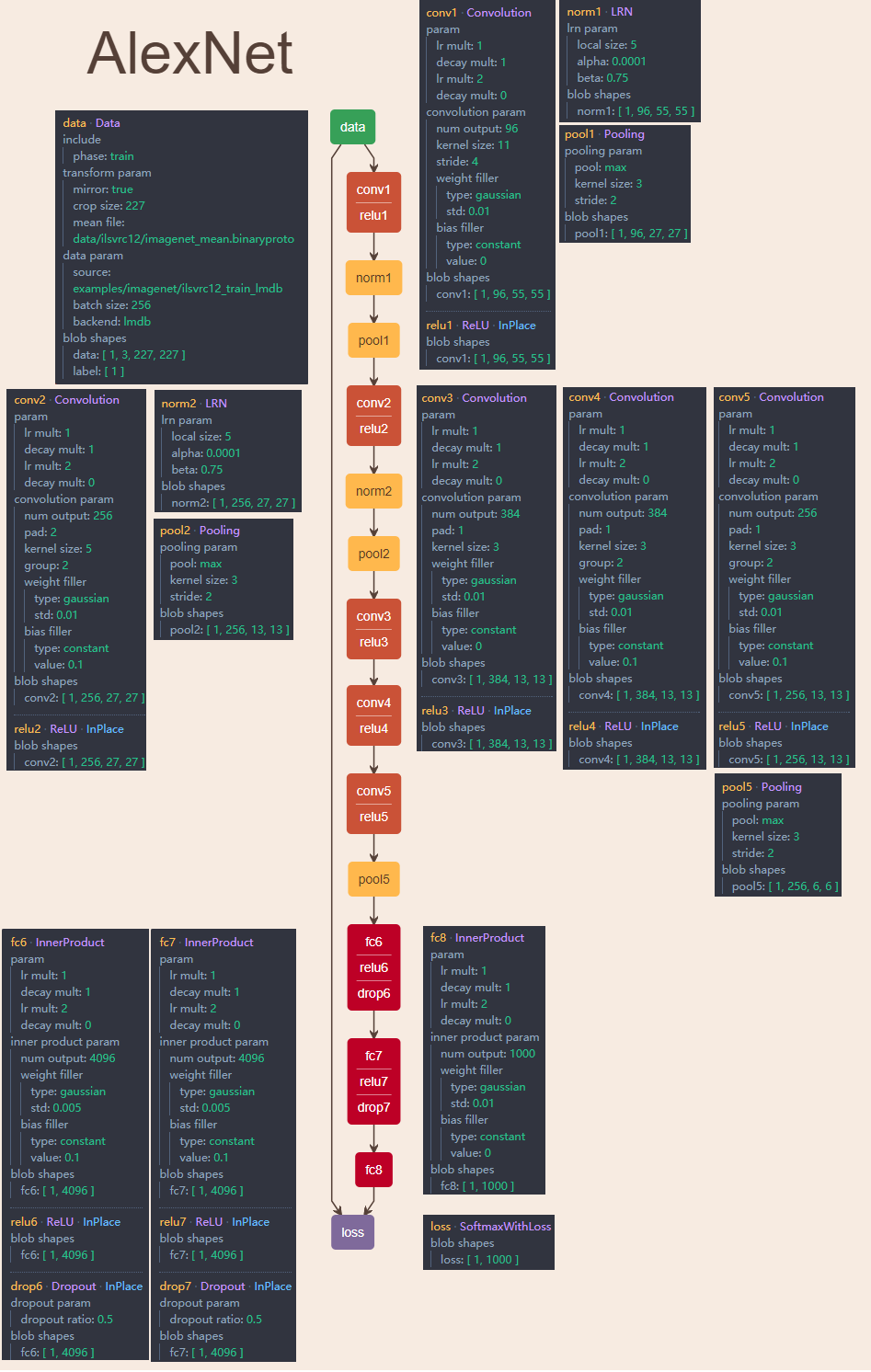### 2.1 ReLU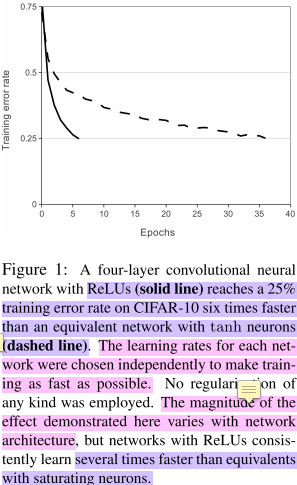### 2.2 多卡训练

This means that, for example, the kernels of layer 3 take input from all kernel maps in layer 2. However, kernels in layer 4 take input only from those kernel maps in layer 3 which reside on the same GPU.### 2.3 Local Response Normalization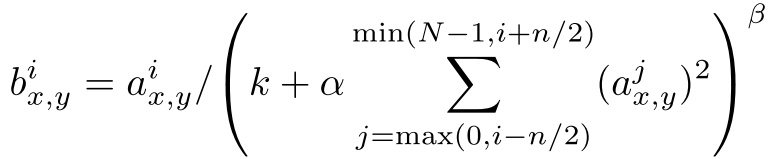template <typename Dtype>
void LRNLayer<Dtype>::Backward_cpu(const vector<Blob<Dtype>*>& top,
const vector<bool>& propagate_down, const vector<Blob<Dtype>*>& bottom) {
switch (this->layer_param_.lrn_param().norm_region()) {
case LRNParameter_NormRegion_ACROSS_CHANNELS:
CrossChannelBackward_cpu(top, propagate_down, bottom);
break;
case LRNParameter_NormRegion_WITHIN_CHANNEL:
WithinChannelBackward(top, propagate_down, bottom);
break;
default:
LOG(FATAL) << "Unknown normalization region.";
}
}

template <typename Dtype>
void LRNLayer<Dtype>::CrossChannelBackward_cpu(
const vector<Blob<Dtype>*>& top, const vector<bool>& propagate_down,
const vector<Blob<Dtype>*>& bottom) {
const Dtype* top_diff = top->cpu_diff();
const Dtype* top_data = top->cpu_data();
const Dtype* bottom_data = bottom->cpu_data();
const Dtype* scale_data = scale_.cpu_data();
Dtype* bottom_diff = bottom->mutable_cpu_diff();
Blob<Dtype> padded_ratio(1, channels_ + size_ - 1, height_, width_);
Blob<Dtype> accum_ratio(1, 1, height_, width_);
Dtype* padded_ratio_data = padded_ratio.mutable_cpu_data();
Dtype* accum_ratio_data = accum_ratio.mutable_cpu_data();
// We hack a little bit by using the diff() to store an additional result
Dtype* accum_ratio_times_bottom = accum_ratio.mutable_cpu_diff();
caffe_set(padded_ratio.count(), Dtype(0), padded_ratio_data);
Dtype cache_ratio_value = 2. * alpha_ * beta_ / size_;

caffe_powx<Dtype>(scale_.count(), scale_data, -beta_, bottom_diff);
caffe_mul<Dtype>(scale_.count(), top_diff, bottom_diff, bottom_diff);

// go through individual data
int inverse_pre_pad = size_ - (size_ + 1) / 2;
for (int n = 0; n < num_; ++n) {
int block_offset = scale_.offset(n);
// first, compute diff_i * y_i / s_i
caffe_mul<Dtype>(channels_ * height_ * width_,
top_diff + block_offset, top_data + block_offset,
padded_ratio_data + padded_ratio.offset(0, inverse_pre_pad));
caffe_div<Dtype>(channels_ * height_ * width_,
padded_ratio_data + padded_ratio.offset(0, inverse_pre_pad),
scale_data + block_offset,
padded_ratio_data + padded_ratio.offset(0, inverse_pre_pad));
// Now, compute the accumulated ratios and the bottom diff
caffe_set(accum_ratio.count(), Dtype(0), accum_ratio_data);
for (int c = 0; c < size_ - 1; ++c) {
caffe_axpy<Dtype>(height_ * width_, 1.,
padded_ratio_data + padded_ratio.offset(0, c), accum_ratio_data);
}
for (int c = 0; c < channels_; ++c) {
caffe_axpy<Dtype>(height_ * width_, 1.,
padded_ratio_data + padded_ratio.offset(0, c + size_ - 1),
accum_ratio_data);
// compute bottom diff
caffe_mul<Dtype>(height_ * width_,
bottom_data + top->offset(n, c),
accum_ratio_data, accum_ratio_times_bottom);
caffe_axpy<Dtype>(height_ * width_, -cache_ratio_value,
accum_ratio_times_bottom, bottom_diff + top->offset(n, c));
caffe_axpy<Dtype>(height_ * width_, -1.,
padded_ratio_data + padded_ratio.offset(0, c), accum_ratio_data);
}
}
}

template <typename Dtype>
void LRNLayer<Dtype>::WithinChannelBackward(
const vector<Blob<Dtype>*>& top, const vector<bool>& propagate_down,
const vector<Blob<Dtype>*>& bottom) {
if (propagate_down) {
vector<bool> product_propagate_down(2, true);
product_layer_->Backward(top, product_propagate_down, product_bottom_vec_);
power_layer_->Backward(power_top_vec_, propagate_down, pool_top_vec_);
pool_layer_->Backward(pool_top_vec_, propagate_down, square_top_vec_);
square_layer_->Backward(square_top_vec_, propagate_down,
square_bottom_vec_);
split_layer_->Backward(split_top_vec_, propagate_down, bottom);
}
}

LRN层 backprop 的计算根据 LRN 的 norm_region 的不同分两种情况：一种是 ACROSS_CHANNELS（会调用CrossChannelBackward_cpu(top ,propagate_down, bottom)），另一种是WITHIN_CHANNEL（会调用WithinChannelBackward(top, propagate_down, bottom)）。压力实在很大，看不下去了，放弃！

void ResponseNormLayer::bpropActs(NVMatrix& v, int inpIdx, float scaleTargets, PASS_TYPE passType) {
convResponseNormUndo(v, _denoms, _prev->getActs(), getActs(), _prev->getActsGrad(), _channels, _size, _scale, _pow, scaleTargets, 1);
}

### 2.4 Overlapping Pooling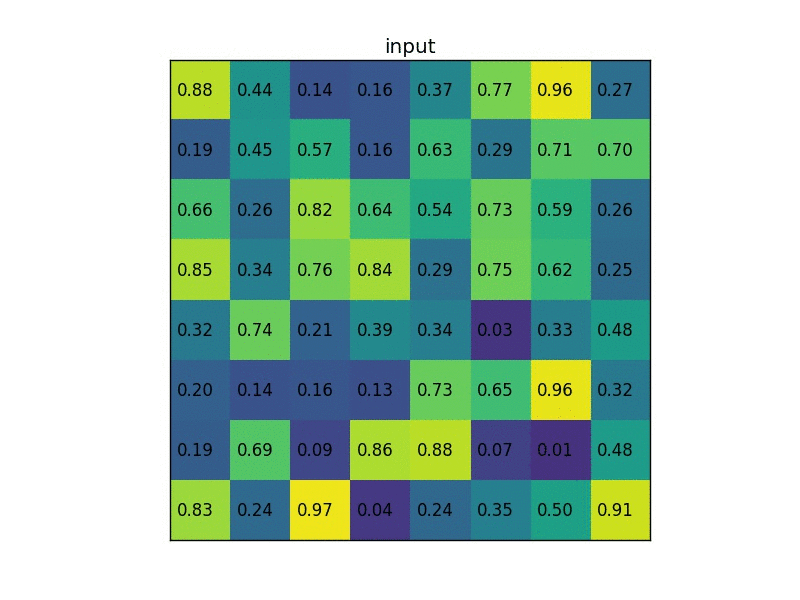We generally observe during training that models with overlapping pooling find it slightly more difficult to overfit.

“有了重叠池化网络不易过拟合”。多少有点反直觉，池化带来信息量减少，重叠形式带来非极大值抑制的作用。直觉上觉得这样会更快收敛，但准确性上不好说（如果作者可以进一步分析一下就更好了）。但实际这里性能的提升相比不带重叠的确实很微弱：top1和top5分别提升0.4%和0.3%。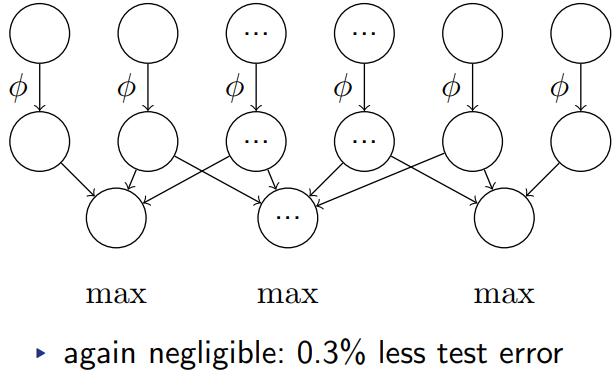• Accepts a volume of size $W1×H1×D1$$W1×H1×D1$
• Requires two hyper-parameters:
• their spatial extent F,
• the stride S,
• Produces a volume of size $W2×H2×D2$$W2×H2×D2$ where:
• $W2=\left(W1-F\right)/S+1$$W2=(W1−F)/S+1$
• $H2=\left(H1-F\right)/S+1$$H2=(H1−F)/S+1$
• $D2=D1$$D2=D1$
• Introduces zero parameters since it computes a fixed function of the input
• Note that it is not common to use zero-padding for Pooling layers

## 3. Reducing Overfitting

Although the 1000 classes of ILSVRC make each training example impose 10 bits of constraint on the mapping from image to label, this turns out to be insufficient to learn so many parameters without considerable overfitting.

• Data augmentation
• Dropout

### 3.1 Data Augmentation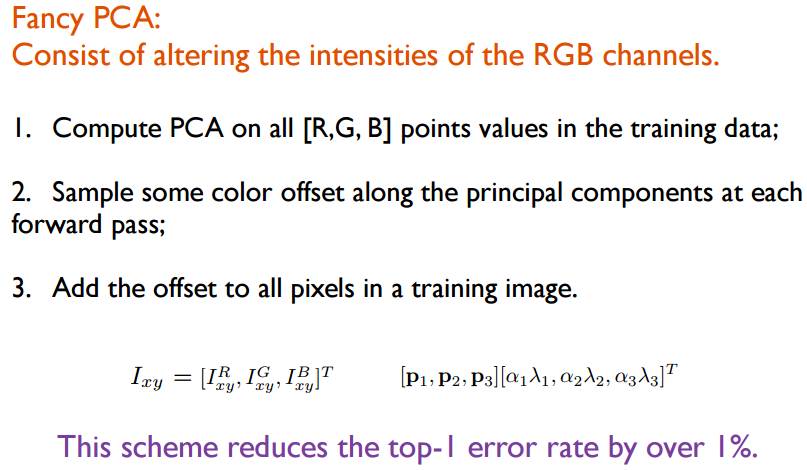### 3.2 Dropout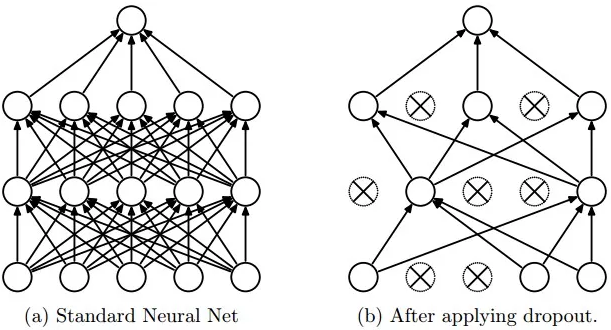dropout解决模型过拟合的问题，但带来的一个问题是 double了 iteration 次数才收敛。实现是通过设定一个dropout的概率dropOutFraction，训练过程中的forward和backward会随机使这一层的神经元以 $p$$p$ 的概率失活，在AlexNet中dropout在前两个全连接层。deeplearn toolbox的实现如下：

% dropout
% forward stage in NN\nnff.m
if(nn.dropoutFraction > 0)
if(nn.testing)
nn.a{i} = nn.a{i}.*(1 - nn.dropoutFraction);
else
nn.dropOutMask{i} = (rand(size(nn.a{i}))>nn.dropoutFraction);
nn.a{i} = nn.a{i}.*nn.dropOutMask{i};
end
end
% backward stage in NN\nnbp.m
if(nn.dropoutFraction>0)
d{i} = d{i} .* [ones(size(d{i},1),1) nn.dropOutMask{i}];
end
• forward：可以看到神经元失活是先随机初始化一个与当前层规模一样的mask，然后根据mask中每个元素的随机值（介于0到1之间），将小于dropoutFraction的元素设置为0，其余为1，得到dropOutMask。再将该层的所有激活值与dropOutMask进行点乘，得到结果。照 deeplearn toolbox的代码显示，每次forward随机的mask都是不同的；
• backward：backprop回来的所在层的derivatives乘以对应层的dropOutMask

test：所有神经元参与计算，但是每个元素值乘以(1-dropOutFraction)，作为没有被dropout的比率被留下的值。

## 4. Details of learning

• 权重初始化：每层权重根据均值为0标准差为0.01的高斯分布初始化，第二、第四、第五层卷积和全连接层的bias初始化为常数1，剩下所有层的bias初始化为0。这种初始化有助于早期阶段正样本的学习的加速；

• 优化算法：小批量梯度下降；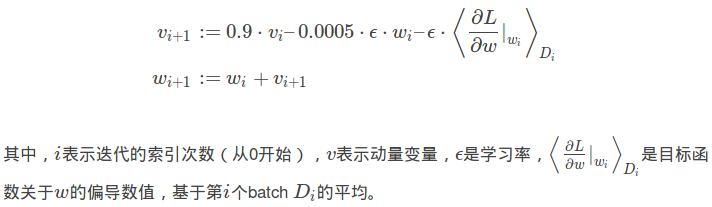• batch size：128。其实这个bsize感觉很大，两个 GTX580 3GB能Cover住，尤其是每张图是224大小的，我表示震惊，这里没具体分析

• learning rate：0.01，所有层使用一样的初始学习率。训练过程中如果val-error不降低，那么就会对learning rate除以10。训练总共跑了90个epochs，3次因为val-error不降低调整学习率；

• momentum：0.9；

• weight decay：0.0005。重要，不仅起正则（提高val-acc）作用，也提高train-acc；

• epochs：90。跑了90遍（两张GTX 580 3GB，跑了5、6天，估算下来230img/sec/2gpus，感觉很挺快的）120万张图像的数据集，前面说到仅仅crop+flip的data augmentation就做了2178倍的数据扩增，这里需要说明的是，data augmentation不是说跑一张图像会跑2178次，而是说每个epoch每张图像都会有2178种随机变化的可能性，使得每次数据有一定的变化。

## 5. Feature Extractor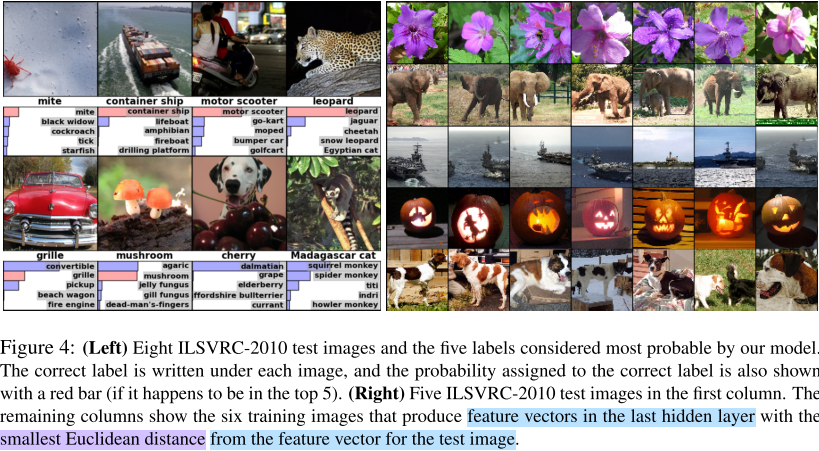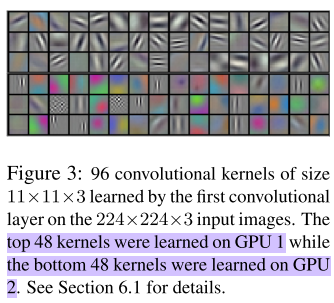## 参考

 ImageNet Classification with Deep Convolutional Neural Networks

 CS231n Convolutional Neural Networks for Visual Recognition - http://cs231n.github.io/convolutional-networks/#pool - http://cs231n.stanford.edu/slides/2017/cs231n_2017_lecture3.pdf

 [IBM Deep Learning “专用” S822LC 服务器深(fu)度(yan)测评 – HPCCHINA] (http://hpcchina.net/ibm-deep-learning-server-s822lc-review/)